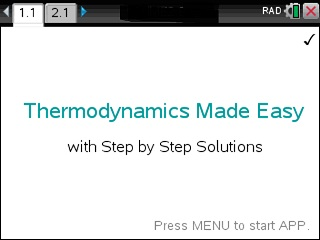## App Purchase

Enter the last 8 digits of your 27-digit TI-Nspire's Product ID.

Located under 5:Settings → 4:Status → About ID may look like: 1008000007206E210B0BD92F455 HELP. If this was your ID you would only type in BD92F455.

At the end of the PayPal checkout, you will be sent an email containing your app. Read Installation InstructionsPrice:\$49.95

## Description

### THERMODYNAMICS

• 1. Law: Δ=Q-W Closed
• 2. Law s2-s1=Δ/t+Sgen Closed
• Read the Laws of Thermodynamics
• Read: Solutions to Maxwell Equations
• Conduction Q=-k*A*(ti-to)/l
• Convection Q=h*A*(Ts-Tf)
• Turbine Efficiency n=(hi-he)/(hi-hes)

### THERMODYNAMICS2

• Heat: Q=m*c*Δ
• Heat: Q=m*L
• W=Δ*d*Δ
• w=-n*r*t*ln(vf/vi)
• w=k*r*(t1-t2)/(k-1)
• H=m*c*T
• Δ=m*c*ln(vf/vi)
• Δ=Δ-n*r*t
• Δ=Δ+n*r*t
• w=-p*Δ
• Cp=q/Δ
• Mass Balance Σ(mi)-Σ(me)=m
• Linear: (k-k1)/(k2-k1)=(u-u1)/(u2-u1)

### ENERGY

• Kinetic: Translational
• Kinetic: Rotational
• E_pot=E_kin : Δ^2=m/k*v^2
• Potential Energy
• Potential: Spring (Us)
• Potential: Universal Gravitat. (UG)
• Miscellaneous: W=F*s*cos(Θ)
• Miscellaneous: W=F*s*sin(Θ)
• Miscellaneous: Power=W/t
• Miscellaneous: E=mu*dx
• Work Energy Theorem
• Photon Energy: E=h*c/lambda

### GAS LAWS

• Volume of Gas
• Boyle's Gas Law
• Charles' Gas Law
• GayLussac Gas Law
• Combined Gas Law
• Ideal Gas Law
• Adiabatic Expansion of Ideal Gas Law
• Gas Sum / Dalton Law
• Graham's Gas Law

• Help & Use of Keys
• What to Input
• Set FontSize

### EXTRAS

• Periodic Table of Elements: Symbol
• Periodic Table of Elements: Element Name
• Find Absolute Error and % Error
• Convert: Normal to Scientific Notation & back
• Density=Mass/Volume Solver
• Moles & Representative Particles
• Atoms to Moles Conversion
• Relativity: Time-Dilation
• Relativity: Mass-Dilation

### SPECIFIC HEATS

• Water
• Ice
• Steam
• Methyl Alcohol
• Benzene
• Wood
• Soil
• Air
• Aluminum
• Marble
• Glass
• Iron/Steel
• Copper
• Silver
• Mercury
• Gold